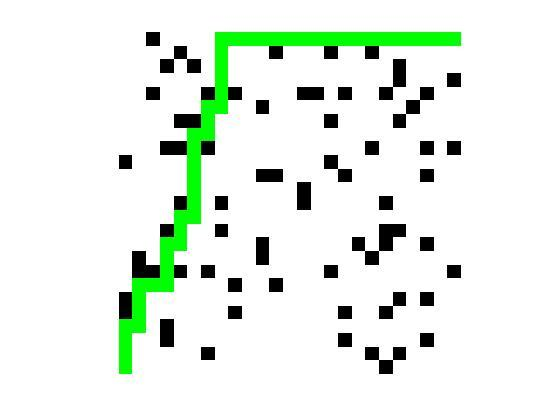## 1 算法特点

Dijkstra使用了广度优先搜索解决赋权有向图或者无向图的单源最短路径问题，算法最终得到一个最短路径树。

## 2 算法的思路

Dijkstra算法采用的是一种贪心的策略，声明一个数组dis来保存源点到各个顶点的最短距离和一个保存已经找到了最短路径的顶点的集合：T。

T初始化为：T={v1}。

## 4 Dijkstra的时间复杂度

Dijkstra算法最简单的实现方法是用一个链表或者数组来存储所有顶点的集合Q，所以搜索Q中最小元素的运算只需要线性搜索Q中的所有元素。这样的话算法的运行时间是## 6 代码

clc;
clear;
close all;
numofobs = length(obs(:,1));
%% set up color map for display
cmap = [1 1 1; ...% 1 - white - 空地
0 0 0; ...% 2 - black - 障碍
1 0 0; ...% 3 - red - 已搜索过的地方
0 0 1; ...% 4 - blue - 下次搜索备选中心
0 1 0; ...% 5 - green - 起始点
1 1 0];...% 6 - yellow -  到目标点的路径
colormap(cmap);
map = zeros(25);
% 设置障碍
for j=1:numofobs
xobs(j) = obs(j,1);
yobs(j) = obs(j,2);
map(xobs(j),yobs(j)) = 2;
end
map(1,25) = 5; % 起始点
map(25,1) = 6; % 目标点
image(1.5,1.5,map);
grid on;
axis image;
%%
nrows = 25;
ncols = 25;
start_node = sub2ind(size(map), 1, 25);
dest_node = sub2ind(size(map), 25, 1);
% Initialize distance array
distanceFromStart = Inf(nrows,ncols);
distanceFromStart(start_node) = 0;
% For each grid cell this array holds the index of its parent
parent = zeros(nrows,ncols);
t0=clock;
% Main Loop
while true
% Draw current map
map(start_node) = 5;
map(dest_node) = 6;
image(1.5, 1.5, map);
grid on;
axis image;
drawnow;
% Find the node with the minimum distance
[min_dist, current] = min(distanceFromStart(:));
if ((current == dest_node) || isinf(min_dist))
break;
end;

map(current) = 3;
distanceFromStart(current) = Inf;
[i, j] = ind2sub(size(distanceFromStart), current);
neighbor = [i-1,j;...
i+1,j;...
i,j+1;...
i,j-1]
outRangetest = (neighbor(:,1)<1) + (neighbor(:,1)>nrows) +...
(neighbor(:,2)<1) + (neighbor(:,2)>ncols )
locate = find(outRangetest>0);
neighbor(locate,:)=[]
neighborIndex = sub2ind(size(map),neighbor(:,1),neighbor(:,2))
for i=1:length(neighborIndex)
if (map(neighborIndex(i))~=2) && (map(neighborIndex(i))~=3 && map(neighborIndex(i))~= 5)
map(neighborIndex(i)) = 4;
if distanceFromStart(neighborIndex(i))> min_dist + 1      distanceFromStart(neighborIndex(i)) = min_dist+1;
parent(neighborIndex(i)) = current;
end
end
end
end
TimeCost=etime(clock,t0)
%%
if (isinf(distanceFromStart(dest_node)))
route = [];
else
%提取路线坐标
route = [dest_node];
while (parent(route(1)) ~= 0)
route = [parent(route(1)), route];
end
% 动态显示出路线
for k = 2:length(route) - 1
map(route(k)) = 7;
pause(0.1);
image(1.5, 1.5, map);
grid on;
axis image;
end
end
axis off;  

## 7 obsdata.txt

10 1 0.5
20 1 0.5
21 1 0.5
17 2 0.5
18 2 0.5
1 3 0.5
5 3 0.5
18 3 0.5
3 4 0.5
9 4 0.5
15 4 0.5
22 4 0.5
23 4 0.5
2 5 0.5
7 5 0.5
9 5 0.5
13 5 0.5
18 5 0.5
3 6 0.5
7 6 0.5
24 7 0.5
5 7 0.5
9 7 0.5
18 7 0.5
13 8 0.5
15 8 0.5
5 9 0.5
19 9 0.5
21 9 0.5
6 11 0.5
11 11 0.5
16 11 0.5
17 11 0.5
2 12 0.5
11 12 0.5
19 12 0.5
5 14 0.5
12 14 0.5
13 14 0.5
5 15 0.5
2 16 0.5
7 16 0.5
10 16 0.5
18 16 0.5
5 17 0.5
11 17 0.5
21 17 0.5
23 17 0.5
16 18 0.5
2 19 0.5
9 19 0.5
17 19 0.5
24 19 0.5
5 20 0.5
13 20 0.5
15 20 0.5
16 20 0.5
21 20 0.5
25 20 0.5
3 21 0.5
4 21 0.5
7 21 0.5
15 21 0.5
20 21 0.5
24 21 0.5
6 22 0.5
5 23 0.5
9 23 0.5
11 23 0.5
16 23 0.5
20 23 0.5
23 23 0.5
4 25 0.5
9 25 0.5
18 25 0.5